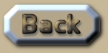Relativity Simulation of Venus

 Relativity and the orbit of Venus I am going to use the orbit of Venus to explain how the orbit-gravity-sim-12.exe (OGS12) algorithm calculates the effect of gravity travelling at the velocity of light from General Relativity. Programming General Relativity is all about determining the direction of the gravity-origin. This gravity-origin will be different from the position of the bodies in an orbital structure due to the velocity of those bodies and the alleged velocity of gravity. Binary systems have a large difference between the position and gravity-origin due to both objects having significant velocity. But even the comparatively small gravity of Venus has an impact on the position of the Sun, oscillating it between 6 or 7 km per orbit at a pedestrian pace of about 86mm per second (which is about the speed of a tortoise in a hurry). Now it takes 6 minutes to travel at the velocity of light from Venus to the Sun. So if gravity travels at the velocity of light, Venus will be pulled to a point about 30m away from the centre of the Sun. (0.086 m/s x 360s = 31m). Of course, the impact that the small gravity of Venus has on the Sun is also delayed. So how do those tiny amounts change the orbit of Venus? Scenario  calculates that according to Newtonian gravity, if Venus is at a distance of 108 940 965.221028km after 1 orbit – then it is: 108 940 965.221242km after 10 orbits – which is an error-margin of: 214mm over 9 orbits (23 mm per orbit). Scenario  calculates that with General Relativity Venus is: 108 940 972.276101km at the 10th orbit– which results in: 7.055 kilometres outward drift, or 784 metres per orbit after 9 orbits - due to gravity moving at the velocity of light. As regards the alteration that Special Relativity would have on Venus, it is very small, because Venus has an orbit that is more than 98% circular. The result is an inward drift of about 40m per orbit, so that in no way cancels out the outward drift of 780m per orbit from General Relativity like so many people believe it should. .This is an extract summary of Chapter XXX of the book: Flight Light and Spin